7-32.

### Missing problem for Book MC2 => Chapter Ch7 => Lesson 7.1.3 => Problem 7-32

Created from orphaned homework help problem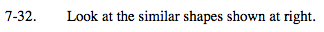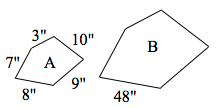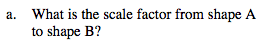What is the factor between 48 and 8?

Multiply by 6.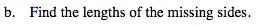Multiply each side from shape A by the scale factor to get the new sides for shape B.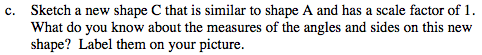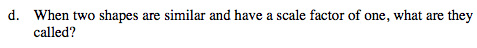Congruent